﻿ Synchronized Clocks and Time on a Rotating Disc

### Synchronized Clocks and Time on a Rotating Disc

Richard LenkOPEN ACCESSPEER-REVIEWED

## Synchronized Clocks and Time on a Rotating Disc

Institute of Physics, Chemnitz University of Technology, Chemnitz, Germany

### Abstract

Basic for the definition of “time” are clocks operating under stationary conditions. In this manuscript synchronized clocks and time on a disc rotating with constant angular velocity are investigated independent of relativity theory. The periods of two clocks can be compared with each other via two return experiments. The central clock mediates between the rotating and the inertial frame. Dimensional arguments and a detailed deduction show that the period of a rotating clock, measured externally in the inertial system, is the same as the internally defined one. There is a common synchron-time, especially “absolute” simultaneity and invariance of flight times hold. This allows a new discussion of the twin problem. The invariance of a rotating circle allows the transference of the inertial polar coordinates to the rotating plane. The flight time of a light pulse obeys a generalized Fermat’s principle. If rays are well defined, this time of flight determines the phase shift of the wave and the result of interference experiments. All general considerations and proofs are independent of the relativity theory, especially of the concept of local inertial systems and differential-geometrical techniques.

• Lenk, Richard. "Synchronized Clocks and Time on a Rotating Disc." International Journal of Physics 1.5 (2013): 115-120.
• Lenk, R. (2013). Synchronized Clocks and Time on a Rotating Disc. International Journal of Physics, 1(5), 115-120.
• Lenk, Richard. "Synchronized Clocks and Time on a Rotating Disc." International Journal of Physics 1, no. 5 (2013): 115-120.

 Import into BibTeX Import into EndNote Import into RefMan Import into RefWorks

### 1. Introduction

As stated in a recent paper , after a century of discussion with a lot of controversies the subject of the physics on a rotating disc “is still alive”. Nearly all contributions are related to relativity theory. A large number of citations can be found in , additionally we quote . Authors engaged in this field have published their different approaches even in a book, cf. the reference . A single paper cannot review this large amount of trials to treat rotation with relativistic methods.

In a strict sense, special relativity theory (SRT) is restricted to the translational motion between two inertial systems. Thus SRT cannot be applied to rotation without additional postulates and/or modifications. Widely used is the concept of local inertial systems, but it cannot explain the experimental results for rotation. Some authors combine the formalism of SRT with postulates that are not in agreement with SRT, especially “absolute simultaneity” is claimed in  and .

SRT is based on the relativity principle (RP). It formulates the symmetry of space- and time-equivalence of inertial systems. A symmetry cannot be broken “only a bit”, and obviously it is broken by rotation. Therefore the present paper starts from a completely different conception. This needs a physical fact that can replace the RP. The existence of a central clock, belonging to both reference systems, is this fact. This clock mediates between the inertial and rotating clocks, and this allows to prove the “invariance of time”. The existence of a common clock is very specific for the rotational problem. Thus the method cannot be applied to other problems, especially not to translation.

Despite of the conceptional difference, a comparison between translation and rotation is given throughout the present paper wherever possible: especially for the (common) internal comparison in Chapt. 2; for the (common) external measurement of periods in the inertial system and for the (different) considerations to compare the internal periods of clocks in relative motion in Chapt. 3; employing in both cases the relativistic concept that the inertial light propagation does not depend on the motion of the source (Chapt. 4). Of special interest is the correspondence for light clocks (Chapt. 7).

In our approach “time” is defined as a primary physical quantity for its own, realized by synchronized clocks. The method is based on the invariant cycle numbers of clocks. The definition of “time” needs the solution of two independent problems: the comparison of periods, and a unique prescription for the simultaneity of distant events. Both problems are solved for inertial systems, usually based on the established inertial symmetries: Clocks at different points in space the same period can be attributed to if they are identical as physical objects; and simultaneity is defined according to the Einstein-synchronisation. In some papers  and  this “gauge” is considered merely as the simplest choice, and anisotropic ones should be equally allowed. In the appendix 1, a possibility is sketched to exclude experimentally the claimed ambiguity. The inertial time is “isotropic” and univocally defined.

### 2. Comparison of Periods

Different processes can be compared with each other only. These comparisons are standardized by clocks. The basic property of a clock is the existence of countable cycles. The numbers ΔN of cycles between two given “moments” are independent of the reference system and of the motion of clocks. Due to the presumed stationarity in time, the same time interval, the period T, can be attributed to each period of a given clock. The periods of two clocks at fixed positions can be compared directly. In two return experiments a signal is emitted from one of the clocks and reflected at the other clock back to the first one where the number ΔNR(i|j) of cycles is counted. For light pulses there is no possibility to choose different signal velocities as for massive particles. Thus the path from 1 to 2 is univocally defined, and that from 2 to 1 too. In the case of rotation, these two paths are different. In both experiments the two pulses travel along these paths in different order only. Due to this equivalence the same time interval T1 ΔNR(1|2) = T2 ΔNR(2|1) belongs to both return processes. The quotient of the “general” periods is thus determined by that of the cycle numbers. The consistency relation (T1/T2)·(T2/T3)·(T3/T1) = 1 can be tested by three independent experiments. There is no need for a metrical analysis of the signal paths. The stationarity in time can be tested: The cycle numbers must remain the same if the experiment is repeated once and again.

The method allows to use different clocks in a given reference system, especially in an inertial system or in a frame rotating with constant angular velocity around a fixed axis, and in a static gravitational field, too. The periods of atomic clocks can be compared there, even combined with a common rotational motion as in GPS  if the gravitational potential acting on each of the clocks remains the same in the course of that motion. If it is not possible to transmit signal pulses directly, as on the surface of earth, a reflector can be placed between.

For a rotating frame all general problems are restricted to planes perpendicular to the axis. On a rotating disc the periods of all clocks C can be especially compared with the period of the central clock Z. For the internally determined period T(int) of a rotating clock one gets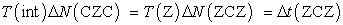(1)

The second equality is valid because the central clock belongs to the rotating and the inertial system as well. Thus Δt is the inertial time difference for the (ZCZ)-process, measured with the central clock.

The central clock belongs to the rotating and the inertial system as well. Thus the clock periods of both systems can be compared independently with the period of this common reference clock and, notwithstanding their relative motion, due to elementary logic also with each other. The mediation via the central clock is a unique aspect of the rotational problem. Methodically it is equally important as the relativity principle for translation. This is decisive for the following considerations.

### 3. Period of Moving Clocks

The return experiment can be also performed with two clocks moving commonly against an inertial system. For translation with constant velocity and for rotation with constant angular velocity, each of the two inertial signal paths is equivalent to the corresponding path at a later time, due to the inertial homogeneity in the first and to the isotropy in the second case. Thus the stationarity in time remains valid, and both moving clocks an externally, i.e. defined in the inertial system, period T(ext) can be attributed to, see below. The quotient of the invariant cycle numbers gives the quotient of these periods in the same manner as that of the T(int). Thus, for any clock, T(ext) ~ T(int) must hold, with a dimensionless factor that depends only on the parameters of the motion. For translation, v/c is this parameter, whereas for rotation there is none. The translational time dilatation is therefore not in conflict with the invariance of T1/T2, whereas for rotation T(ext) = T(int) is the only possibility.

For a single moving clock C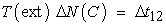(2)

is the definition of T(ext) where Δt12 is the time difference between those two synchronized inertial clocks that the moving clock passes at the beginning and at the end of the measurement process not yet specified. For eq. (2) to be meaningful, however, the necessary homogeneity and isotropy, see above, must not be destroyed by the prescription for the setting of clocks. The Einstein-synchronization meets this demand.

For a rotating clock, the measurement process in eq. (2) can be specified to a return process via the central clock, ΔN(C) → ΔN(CZC). The comparison of eq. (2) with eq. (1) yields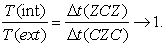(3)

In the (ZCZ)-process, the moving clock acts only once (as “reflector”), whereas twice (as “source” and as “detector”) in the (CZC)-process. As already discussed, due to the inertial isotropy the pulses travel along equivalent paths, and the two time differences in eq. (3) are equal. In accordance with the dimensional argument, T(int) = T(ext) holds. This statement can be tested by separate measurements in both reference systems.

If all the inertial clocks have the same period T, i.e. Δt12 = T ΔN12, and the rotating clocks are regulated via the central clock to achieve the same period, eq. (2) yields ΔN(C) = ΔN12: Such a clock “ticks” exactly so as the inertial clocks passed. This is also true for a full circuit. Generally, however, a regulated rotating clock is no more a twin of the inertial ones, and a non-regulated clock my have another period as its twin.

For the translational case, the period of a moving clock shows time dilatation TD as compared with the twin-clocks at rest. This general result follows from the relativity principle and holds if the twin criterion is correct. There is no problem with the latter for quantum objects. – For rotating clocks, especially for atomic clocks, TD is often assumed, based on the concept of local inertial systems. This may be true; there is no relationship to the invariance of the period in the sense of eq. (3).

### 4. Common Time

With the invariance eq. (3) of the period, eq. (2) reads simply Δt(rot) = Δt(inert) for the time difference between two arbitrarily chosen events. If one of the rotating clocks is set, only once, to indicate the same time-value as the inertial clock just passed, this equality of times remains in the further course of motion: Each event the same time is attributed to in both reference systems. Especially, two events are simultaneous in the rotating system if they are so in the inertial one. The inertial consistency and univocality of the simultaneity of distant events is thus transferred to the rotating system. The setting of each clock described above amounts to an external prescription for the synchronization of the rotating clocks, see the next paragraph for the internal condition.

With common unit of time and “absolute” simultaneity, a common synchron-time is defined. The clocks of both systems realize this time; they constitute a single ensemble. This time concept is completely independent of a metric in space. “Common time” fits the success of GPS  where the synchron-time TAI on the rotating earth is matched with the time of the satellites rotating against distant stares.

In , the author postulates “absolute simultaneity” and combines it with differential-geometrical methods in the spirit of SRT. Relativistic time dilatation remains, however. In  absolute simultaneity is introduced to explain the Sagnac effect.

With common time, flight times of signal pulses can be determined in the inertial system for a rotating source-detector configuration and employed without change in the rotating system. If these times are measured, arbitrary signals are allowed. Are they to be calculated, the motion of the source must be considered. Generalizing the translational case, the independence of the inertial light propagation of that motion will be presumed for a rotating source, too. This statement is the essential “relativistic element” in our approach.

### 5. Internal Criterion of Simultaneity

Two light pulses are emitted simultaneously in different directions from two sources at r1 and r2. In the inertial system these pulses meet each other at all points with the same distance from r1 and r2, i.e. in two dimensions on the orthogonal mid-straight. At an arbitrarily chosen point of this line, the two pulses meet at a definite time. This defines a physical event that can, on principle, identified in any reference system. According to the common time, such a point defines especially the simultaneity on the rotating disc. This prescription is independent of a spatial metric.

For practical reasons, and in order to get quantitative results, it is useful to define in the inertial system a suitable registration line RL. In the course of its rigid rotation this line cuts the meeting straight at a special point that can be identified on the rotating plane. Its position can be described mathematically in a polar coordinate system, see the next paragraph. The simple results are already used in the following.

For a radial configuration the two sources are on the same rotating radial stick at distances r1 and r2 from the centre. This stick can be taken as RL, and geometry of plane triangles yields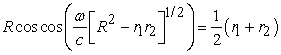(4)

for the position of the simultaneity point. The special case r1 = 0 is sufficient for the setting of all clocks via the central one.

For an azimutale configuration, the two sources define a rotating circle segment and the angle difference Δφ between. The radial straight at Δφ/2 is the meeting line, and the segment itself is a suitable RL. Each of the two pulses flights along its inertial secant, length l, and both meet the rotating RL after the flight time l/c at the position Δφ/2 – ωl/c.

In the three-dimensional case, the inertial Einstein-criterion holds for all points of the axis, and each of these points is the centre of a rotating plane as discussed above – For two sources on a sphere, their positions define

a) an “orthogonal” meeting plane that cuts the sphere on a non-rotating circle and

b) the segment of a circle that connects the sources. This rotates about the given axis.

The common point of both circles defines simultaneity.

### 6. Polar Coordinates

As shown in the first paragraph, the periods of rotating clocks can be compared directly with each other and, via the central clock, even with the periods of the inertial clocks. There is no equivalent possibility for the length of metric rods. Therefore, the definition of rotating lengths is to some extend a matter of choice. This is especially true for the often assumed and discussed  and  azimutale length contraction if there is no independent definition of lengths in the rotating system. For “time” has been defined as independent of a spatial metric, the problem of length contraction is irrelevant in this context.

Times of flight will be calculated with polar coordinates r and φ in the inertial system. To transfer these times to the rotating plane, it is necessary to define there coordinates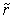and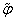in such a manner that no measurements of lengths on this plane are involved. This can be done in the simplest way. Without any markings, a rotating circle, a material ring, cannot be distinguished from one at rest. Its radius r and its circumference U can be measured directly, i.e. without clocks, with the inertial metric rods, and the results are obviously independent of its rotation. Thus U = 2 πr, and the full plane angle is 2π. All these circles constitute concentric marking lines that belong to the inertial and the rotating plane as well, and= r is the best choice, of course. Thus circular marking lines replace radial rotating rods.

Each of the circles can be divided in an arbitrary number n of equal segments. This can be done without measurements of lengths with the same method in the inertial or in the rotating plane. According to the rotational invariance of the circle,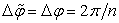is the best definition of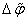. To define φ oritself, a radial straight φ = 0 or= 0, respectively, is needed in the inertial or rotating plane to “synchronize” the division marks between the circle segments. The straight= 0 can be realized as the “projection” of a straight rotating in the inertial plane. The straightness of such a line can be tested by simultaneous registration of a sufficiently large number of points on it. This needs synchronized clocks, and the result depends on the setting of the clocks. This problem has been already discussed, and arguments for isotropic synchronization are given.

With the polar coordinates in the rotating plane defined, the coordinate transformation is given by the invariance of r and by φ → φ - ωt where t is the common time. All relativistic effects are taken into account by the synchronization of the rotating clocks. Especially the free motion of a massive particle is “classical”, influenced by Coriolis and centrifugal force.

### 7. Radial Configuration: Time of Flight, Light Clocks and the Twin Problem

Source and detector are fixed on the same rotating radial stick, one at r1 and the other at r2. The straight path that the light pulse travels along in the inertial systems is the third side in the triangle with sides r1, r2 and the angle ωl/c between them. If the source or the detector is at the centre, one gets l = r for the length of the light path. For slow rotation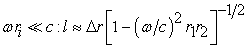(5)

holds. This corresponds with the perpendicular configuration in the case of translation, the geometrical mean of the rotational velocities replaces v. The time of flight l/c can be measured in the rotating system.

In a light clock, the pulse is reflected back and forth between two mirrors. The local character of such a clock needs a small mirror distance, Δr « r1r2. This justifies the same approximation as for slow rotation with the result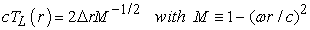(6)

A regulated light clock with the smaller mirror distance Δr = M1/2 Δr(Z) has the same period as the central clock Z.

A radial light-clock is a twin of the inertial light-clocks if the invariant mirror distance Δr is the same. 2Δr/c = T is the period of the inertial twins, and TL(twin) = T M -1/2 > T is the period of the rotating twin which thus behaves in accordance with the so-called twin-paradox. This, however, is not at all strange because the rotating twin does not belong to the ensemble of clocks with the same period. - According to the calculation above, TL(r) is first the externally, i.e. with the synchronized inertial clocks, measured period TL(ext) of the rotating clock as defined in (2). This gives with T(ext) → TL(twin) and ΔNC → ΔNL(twin), the relation ΔNL = M 1/2ΔN12 : the moving twin ticks more slowly than the inertial ones passed. The invariance TL(ext) = TL(int) yield the invariance of the time differences for any number of cycles; thus the concept of common time is reproduced.

For translational motion, ωr has to be replaced by v and Δr by the perpendicular mirror distance. With these replacements, the relations for TL(ext) and ΔNL are the same as for the rotational case. There is full equivalence in this respect; a rotating light clock behaves in accordance with the concept of local inertial systems. There is, however, no way to compare directly the period of the moving clock with the clocks at rest. Instead of the invariance of periods, according to the relativity principle the same period T is attributed to the moving twin in the co-moving inertial system as to the twins at rest. The invariant cycle number of the moving twin thus gives the time difference in the co-moving system if multiplied with T, and, if multiplied with TL(twin), the time difference in that inertial system where the twin is moving. This is the time dilatation already mentioned.

Despite the equivalence of translation and rotation for TL and ΔNL, this result is combined with different theoretical concepts: {inequality T(int) → TT(ext) and time dilatation} for translation, but {equality and common time} for rotation. The symmetry formulated by the relativity principle is broken by the rotation.

### 8. Time of Flight for a General Configuration

The method is the same as already applied to the special radial configuration. Source S and detector D rotate commonly, on two circles with radii r1 and r2, but now with a non-zero angle difference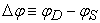, counted as positive in the direction of rotation. The pulse travels along a straight, and its length l is the third side in the triangle with sides r1, r2 and the angle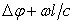. For small values of Δr = r2r1 and Δφ, i.e. ωΔr « c and |Δφ| « 1, one gets with Δl = cΔt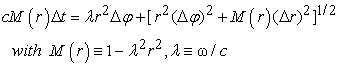(7)

For all physically allowed radii,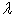r < 1 holds. The sign of the square root is always positive.

The linear term in (7), with Δφ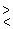0, reflects the inequivalence of pulse motion with or against rotation, often formulated as a difference of the velocity of light. This is the essence of the Sagnac effect, discussed in many papers, see  for a review, with different theoretical conceptions. Without terms of second order in ωr/c and generalized for the surface of the earth, the linear term is included in the definition of TAJ, the International Atomic Time.

With the calculated flight times the setting of clocks is experimentally simpler compared with the time-free (basic) method of meeting points. The light pulse between two clocks can transmit the information of the emission time.

The method explained above to define polar coordinates on the rotating disc is difficult to realize experimentally, and the idea of a “projection” cannot be generalized to three dimensions. These problems can be overcome if the given flight times are, vice versa, used to define the coordinates. A circle of radius r, for example, is given by all points where light pulses emitted from the centre at time t0 arrive at time t0 + r/c. This method can be generalized, especially it applies to the three-dimensional case, too. This possibility underlines the importance of an independent time concept.

### 9. Extremal Principle

The light pulse shall travel along an arbitrarily chosen curve φ = φ(r) from one point 1 to a second point 2, both fixed on the rotating plane. The corresponding time Δt12 can be calculated by integration along this curve,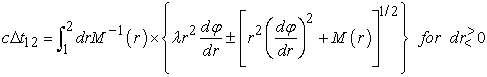(8)

The real path, under the condition of free propagation, is determined by the principle of shortest travelling time. φ is a cyclic variable, and the variation yields the differential equation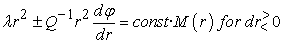(9)

where Q is the positive square root in (8). The integration constant is determined by the end points.

In the inertial system, the real path is a straight line. The transformation φ → φ - ωt gives the curved path wanted. If the function φ(r) thus constructed is a solution of the differential equation, the real path obeys the extremal principle. In the very simple special case r1 = 0 or r2 = 0, the inertial path is a radial straight outwards or inwards, thus φ(inert) = const and dr = ± cdt. This gives dφ/dr =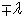and Q = 1 in the rotating system, and the differential equation is fulfilled with const = 0. The general proof is sketched in appendix 2. It supports the concept of common time.

### 10. Remarks on Frequency and Phase of Light Waves

Despite of spatial inhomogeneity and anisotropy, the frequency f of light, emitted by a source at rest in the rotating system, should be the same everywhere. Equivalent to this statement, there is no Doppler effect in the inertial system for a common rotation of source and detector.

Like the period of a rotating clock, the frequency of a source or a resonant absorber is generally not the same as those of the inertial “twins”. These frequencies are to be measured. Resonant absorption in Mößbauer experiments  should be perturbed if source and absorber are at different distances of the centre. For a central source, the frequency is not changed by the rotation.

For short wave lengths, a bundle of rays can be attributed to the wave field. For a point-source S, a flight time Δt(PS) is defined along the ray from S to any point P. The extremal property of Δt renders sensible the thesis that the field at P, as the result of a constructive interference of elementary waves (in the sense of the Huygens-Fresnel principle), oscillates with the phase difference 2πf Δt(PS), thus u(t) ~ exp[2πf(t - ΔtPS)]. With this representation of the wave field, inhomogeneity and anisotropy of the propagation process are taken into account compactly by the time of flight. For example, the condition of periodicity for a resonator reads fnt(12) + Δt(21)] = n. For a ring one gets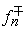T± = n where T± is the flight time around, either with or against the rotation. If relativistic corrections of order (ωr/c)2 can be neglected, the linear term in (7) yields the contribution ± 2ωA/c where A is the area surrounded. This gives, generalized to a sphere, the frequency of a ring laser, especially the difference f+ - f- . The measurement of this difference  and  yields a precise sensor for the earth rotation. The theoretical result of a “common time” has thus been already anticipated from the experimental side.

For two paths from the source to the detector the difference of the flight times determines the intensity in the interference experiment. A suitable parameter can be adjusted to keep maximum intensity for varying angular velocity.

### Appendix 1: Inertial Isotropy of Light Propagation and Test of Simultaneity Criteria

Time differences, especially times of flight, obey (trivially) cyclic zero-relations for three or more events. These relations remain true if to the Δt(ij) terms Δ(ij) are added that depend only on the positions of the events in a projective manner, compare the Lorentz transformation. This yields anisotropy and changes the definition of simultaneity. Sums of flight times, as in ring processes or in coincidence experiments with arbitrary reflex points between source and detector, remain unchanged in the first example or get a constant additive in the second one. Thus anisotropy cannot be excluded that way.

Differences of flight times determine the lines where two pulses, emitted flash-like from two sources, meet each other. For all points of such a line, Δt(ij) has the same value. In the case of isotropy, these lines are hyperbolas with the sources as focal points. Each non-trivial change of the flight times yields other geometrical forms. Thus anisotropy could be detected without clocks and times.

The analysis of meeting lines needs the definition of coordinates in space. A corresponding metric can be useful, it is, however, not necessary. The information coded in the meeting lines should be sufficient to determine flight times univocally, and thus the simultaneity of distant events, too. This statement is not restricted to inertial systems.

### Appendix 2: Proof of the Extremal Principle

For a straight line in the inertial system, the equation r(φ) = A/cos φ holds. A = min r defines the radial ray with φ = 0. For dφ > 0, the pulse moves “with the rotation”. x = r sin φ is the co-ordinate along the straight, thus dx/dt = +c. From the above relation one gets the geometrical relations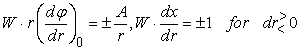(10)

with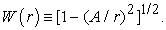For the path in the rotating plane follows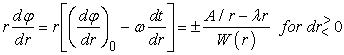(11)

Now the square root Q defined in the text after (9) can be evaluated analytically with the result Q = W-1(1 - λA), and the differential equation is fulfilled with const = A[1 - λA]-1, λA < 1.

For dφ < 0, the sign of the (A/r)-term is changed, and the integration constant takes the value –A[1 + λA]-1.

### References

  Kassner, K., “Spatial geometry of the rotating disk and its non-rotating counterpart”, Am. J. Phys. 80(9), 772, 2012.In article CrossRef  Klauber, R.D., “Toward a Consistent Theory of Relativistic Rotation” in Relativity in Rotating Frames, Fundamental Theories of Physics 135, 103, 2004.In article  Anderson, R., Vetharaniam, I., and Stedman, G.E., “Conventionality of synchronisation, gauge dependence and test theories of relativity”, Phys. Rep. 295, 93, 1998.In article CrossRef  Minguzzi, E., “On the Conventionality of Simultaneity”, Found. Phys. Lett. 15, 153, 2002.In article CrossRef  Ashby, N., Relativity and the Global Positioning System”, Phys. Today, May 2002, 41.In article CrossRef  Selleri, F., “Sagnac effect: end of the mystery”, in Relativity in rotating frames, ed. by G. Rizzi and M.L. Ruggiero, Kluwer Academic Publishers, Dordrecht, 2003.In article  Gron, O., “Relativistic description of a rotating disk”, Amer. J. Phys. 43(10), 869, 1975.In article CrossRef  Champeney, D.C., Isaak, G.R., and Khan, A.M., “A time dilatation experiment based on the Mössbauer effect”, Proc. Phys. Soc. 85, 583, 1965.In article CrossRef  Schreiber, K.U., Klügel, T., Velikoseltsev, A., Schlüter, W., Stedman, G., and Wells, J., “The Large Ring Laser G for Continuous Earth Rotation Monitoring“, Pure and Applied Geophysics 166, 1485, 2009.In article CrossRef  Schreiber, U., “Ein Ring, die Erde zu finden“, Physik Journal 12(5), 25, 2013.In article